## 个性与品质 MINI CLUBMAN进口车型推荐

2014年11月15日 15:35 来源：www.chextx.com 超过：次关注

虽然我们可以将MINI划分到小众车的行列，但是在路上它的出现频率却颇高，原因很简单，经典的复古设计融入了大量的时尚元素，在现在这个就怕撞衫的年代MINI受到了很多消费者的青睐，不仅仅是年轻的小姑娘，还有男性以及阿姨。

就像时装的复古风一样，不知从什么时候起汽车的设计也开始走复古路线，一走就是几年并且依然受到追捧；MINI的产品线比较丰富，价格也是从20万跨越到了40万，但是其外观都保持了一贯的精致复古造型；其实MINI在同界别小型车中似乎找不到直接的竞争对手，哪怕是刚刚上市的奔驰A级也与它不能形成直接的对垒，因为A级更加平和，更照顾的是家用，而MINI要的就是与众不同的张扬，MINI在路上颇为常见，外观设计相信没有人不认可，所以对于外观就不做具体描述了。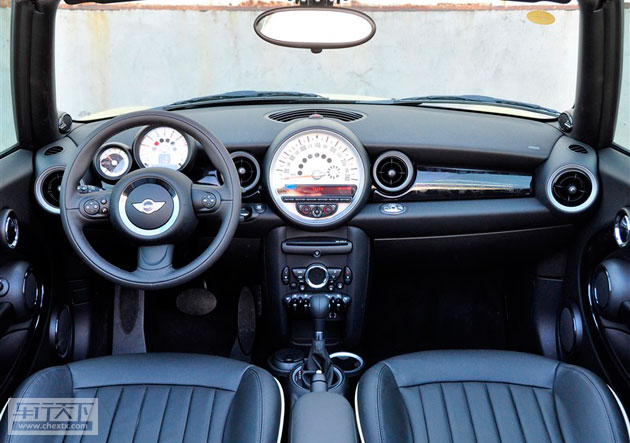MINI的杀伤力绝不仅仅来自于外观，车内的复古的个性设计丝毫不减，内饰部分很大一部分都用圆形处理，仔细从图中观察，后视镜、空调出风口甚至连油门刹车踏板都向圆形靠近；尤其是中置的超大白色仪表盘极具个性；个性不仅局限于此，空调控制区巧妙的设计成了MINI车标的造型，算是增加专属感？另外为了凸显运动以及提升驾驶乐趣，方向盘拨片在“COOPER S”合理的出现。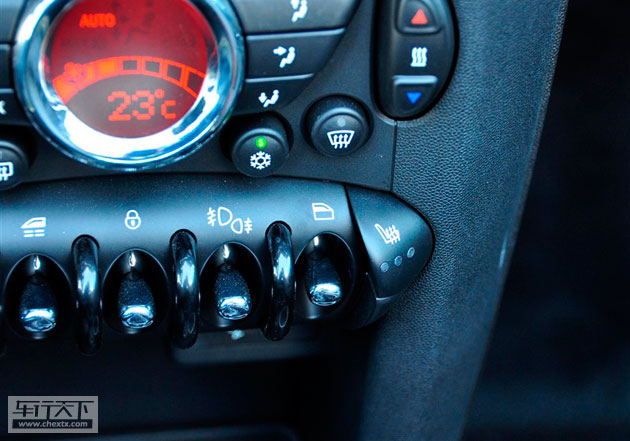MINI全系均为无匙启动，圆形的钥匙造型别致，插入车内的钥匙孔后按动启动按钮即可着车；座椅加热这一项在冬季颇为使用的配置在MINI家族中虽不是全系标配，但是仅有两款入门级车型没有；不过理性的想一想如果仅仅为个性买单是不是有点不值呢，20多万的车带有这些配置在我看来也是理所当然的。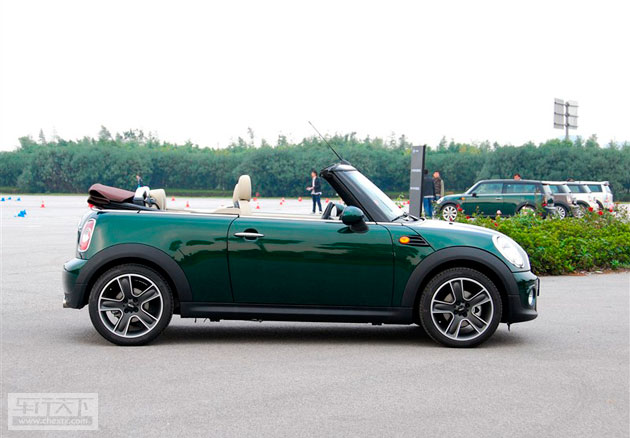想怎么看星星？全景天窗还是干脆敞篷？MINI丰富的产品线可以满足不同人对于生活情调的要求，双天窗的设计令车内可以得到很好的采光或者换气，一款并不大的小型车配备全景天窗的确别具情趣，也算是对后排乘客的一个弥补，如果还不够彻底，干脆选择软顶敞篷。当然这些都是要资金的支持，否则你抬头仅能看见密闭的车顶。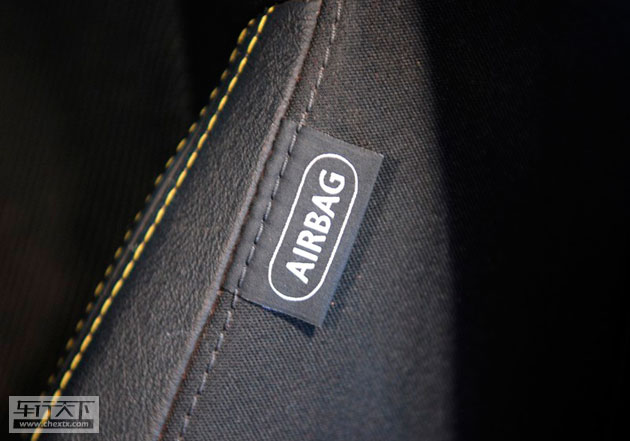在主被动安全方面MINI同样表现出了应有的实力，前排侧气囊以及前后头部气帘，在碰撞之中最大程度的保护车内的乘员，减轻由于碰撞带来的伤害；ESP在车辆即将发生危险动作的时候可以有效的对车身进行必要的修正，减小发生事故的概率。到此不得不希望我们自主品牌或者合资品牌在这些细节上也进行提升，车不是不可以贵，但是要贵的有道理，不能价格不变却眼看着配置和品质下滑。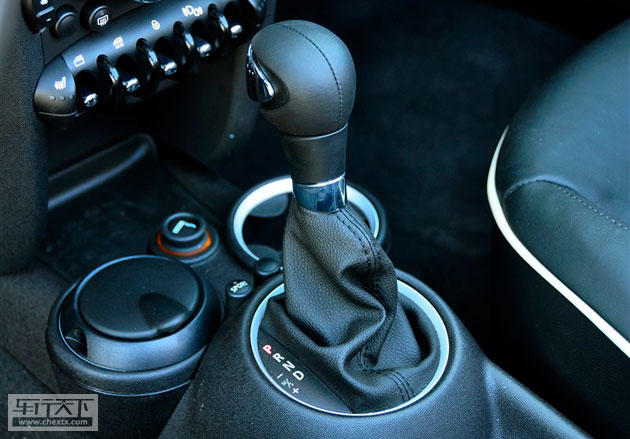MINI都为1.6L但是动力系统却不尽相同，入门级的“one”从配置到动力均比较薄弱；而“COOPER S”则搭载了1.6T增压发动机，最大240牛米的扭矩在这款小车上的表现可想而知；其余车型的1.6L发动机动力同样够用；三个不同方向的动力系统也划分出了不同的定位。

仅仅有复古运动的外观是不能将运动体现出来的，MINI由6挡手自一体变速箱与发动机配合，在小型车甚至紧凑型车中使用6挡变速箱的车型还是比较少见的，紧密的齿比动力传输效率高；在强调动力的同时操控性也是MINI所追求的，后多连杆独立悬挂在舒适和操控上都要强于同级车。

MINI COOPER S是车系中最具个性的车型，有着时尚的外观并且还搭载了一颗强劲的心，我们对其进行实际的动态测试，1.6T发动机推动这台小车在7.2秒内从静止加速到100kM/h，可以称之为钢炮级选手，所以这款车绝不是花瓶一个。

MINI可以说是小型车市场中极具个性的一款时尚座驾，看上去更像是一款玩具；但是继承了宝马家族的技术与到位的操控调教，使得这样一辆小型车在同级别车型中运动的优势明显，与刚刚上市的奔驰A级相比，两款车分别指向了年轻时尚一族和温馨家用一派，互相并不冲突。

﻿
• 快速找车
• 选择品牌
• 选择品牌
• A  奥迪
• A  阿斯顿·马丁
• A  阿尔法·罗密欧
• B  宝沃
• B  布加迪
• B  巴博斯
• B  保时捷
• B  宾利
• B  奔驰
• B  宝马
• B  本田
• B  别克
• B  标致
• B  比亚迪
• B  宝骏
• B  北汽制造
• B  北汽新能源
• B  北汽幻速
• B  北汽威旺
• B  北京汽车
• B  奔腾
• B  北汽绅宝
• B  北汽昌河
• C  长安欧尚
• C  长安
• C  长安凯程
• C  长城
• D  大众
• D  道奇
• D  DS
• D  东南
• D  东风风神
• D  东风风行
• D  东风小康
• D  东风风度
• D  东风
• F  福特
• F  丰田
• F  菲亚特
• F  法拉利
• F  福田
• F  福迪
• F  福汽启腾
• G  观致
• G  广汽传祺
• G  广汽吉奥
• G  GMC
• H  红旗
• H  汉腾汽车
• H  哈弗
• H  哈飞
• H  海格
• H  海马
• H  华颂
• H  黄海
• H  华泰
• H  恒天
• J  几何汽车
• J  捷达
• J  吉利汽车
• J  捷豹
• J  Jeep
• J  江淮
• J  江铃
• J  金杯
• J  九龙
• J  金旅
• K  凯翼
• K  凯迪拉克
• K  克莱斯勒
• K  科尼塞克
• K  卡威
• K  开瑞
• L  路虎
• L  林肯
• L  劳斯莱斯
• L  兰博基尼
• L  雷克萨斯
• L  铃木
• L  领克
• L  雷诺
• L  理念
• L  力帆
• L  莲花汽车
• L  猎豹
• L  路特斯
• L  陆风
• M  马自达
• M  MG
• M  MINI
• M  玛莎拉蒂
• M  摩根
• M  迈凯轮
• N  纳智捷
• O  欧拉
• O  欧宝
• O  讴歌
• O  欧朗
• Q  奇瑞
• Q  起亚
• Q  启辰
• R  日产
• R  荣威
• R  瑞麒
• S  SERES赛力斯
• S  三菱
• S  斯威汽车
• S  萨博
• S  smart
• S  斯柯达
• S  斯巴鲁
• S  思铭
• S  双龙
• S  上汽大通
• S  双环
• T  特斯拉
• T  腾势
• W  蔚来
• W  沃尔沃
• W  WEY
• W  五菱汽车
• W  五十铃
• W  威兹曼
• W  威麟
• X  现代
• X  雪佛兰
• X  星途
• X  雪铁龙
• X  小鹏汽车
• X  西雅特
• Y  一汽
• Y  英菲尼迪
• Y  英致
• Y  依维柯
• Y  野马汽车
• Y  永源
• Z  众泰
• Z  中华
• Z  中兴
• Z  知豆
• 选择车系
• 选择车系
• 车型对比
• 选择品牌
• 选择品牌
• A  奥迪
• A  阿斯顿·马丁
• A  阿尔法·罗密欧
• B  宝沃
• B  布加迪
• B  巴博斯
• B  保时捷
• B  宾利
• B  奔驰
• B  宝马
• B  本田
• B  别克
• B  标致
• B  比亚迪
• B  宝骏
• B  北汽制造
• B  北汽新能源
• B  北汽幻速
• B  北汽威旺
• B  北京汽车
• B  奔腾
• B  北汽绅宝
• B  北汽昌河
• C  长安欧尚
• C  长安
• C  长安凯程
• C  长城
• D  大众
• D  道奇
• D  DS
• D  东南
• D  东风风神
• D  东风风行
• D  东风小康
• D  东风风度
• D  东风
• F  福特
• F  丰田
• F  菲亚特
• F  法拉利
• F  福田
• F  福迪
• F  福汽启腾
• G  观致
• G  广汽传祺
• G  广汽吉奥
• G  GMC
• H  红旗
• H  汉腾汽车
• H  哈弗
• H  哈飞
• H  海格
• H  海马
• H  华颂
• H  黄海
• H  华泰
• H  恒天
• J  几何汽车
• J  捷达
• J  吉利汽车
• J  捷豹
• J  Jeep
• J  江淮
• J  江铃
• J  金杯
• J  九龙
• J  金旅
• K  凯翼
• K  凯迪拉克
• K  克莱斯勒
• K  科尼塞克
• K  卡威
• K  开瑞
• L  路虎
• L  林肯
• L  劳斯莱斯
• L  兰博基尼
• L  雷克萨斯
• L  铃木
• L  领克
• L  雷诺
• L  理念
• L  力帆
• L  莲花汽车
• L  猎豹
• L  路特斯
• L  陆风
• M  马自达
• M  MG
• M  MINI
• M  玛莎拉蒂
• M  摩根
• M  迈凯轮
• N  纳智捷
• O  欧拉
• O  欧宝
• O  讴歌
• O  欧朗
• Q  奇瑞
• Q  起亚
• Q  启辰
• R  日产
• R  荣威
• R  瑞麒
• S  SERES赛力斯
• S  三菱
• S  斯威汽车
• S  萨博
• S  smart
• S  斯柯达
• S  斯巴鲁
• S  思铭
• S  双龙
• S  上汽大通
• S  双环
• T  特斯拉
• T  腾势
• W  蔚来
• W  沃尔沃
• W  WEY
• W  五菱汽车
• W  五十铃
• W  威兹曼
• W  威麟
• X  现代
• X  雪佛兰
• X  星途
• X  雪铁龙
• X  小鹏汽车
• X  西雅特
• Y  一汽
• Y  英菲尼迪
• Y  英致
• Y  依维柯
• Y  野马汽车
• Y  永源
• Z  众泰
• Z  中华
• Z  中兴
• Z  知豆
• 选择车系
• 选择车系
• 选择车型
• 选择车型
• 意见反馈